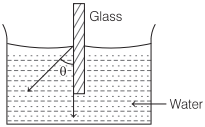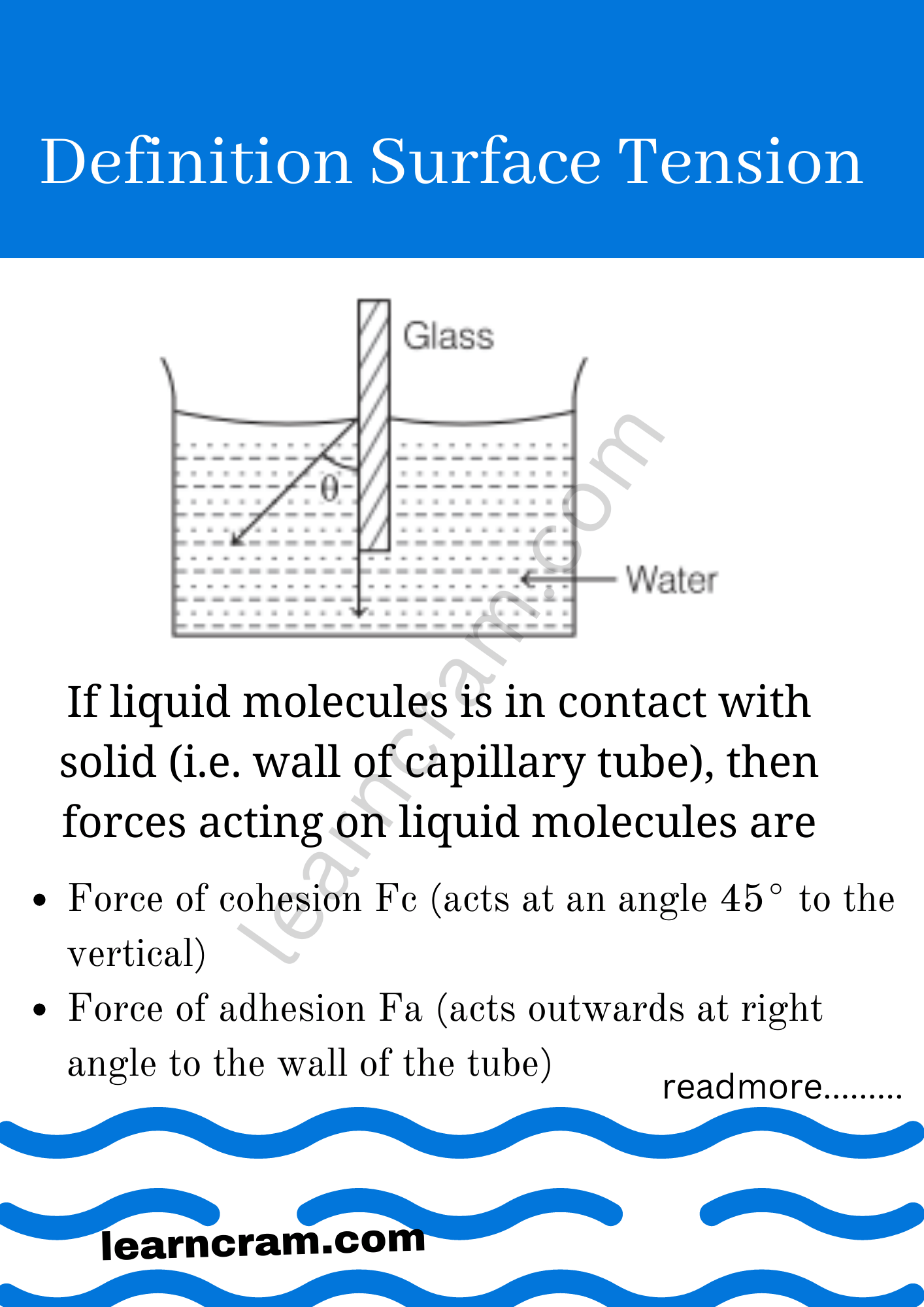# Angle of Contact in Surface Tension Definition, Formula and Examples – Physics

Define Angle of Contact:
The angle subtended between the tangents drawn at liquid surface and at the solid surface inside the liquid at the point of contact is called angle of contact (θ).

We are giving a detailed and clear sheet on all Physics Notes that are very useful to understand the Basic Physics Concepts.

## Angle of Contact in Physics | Definition – Surface Tension

If liquid molecules is in contact with solid (i.e. wall of capillary tube), then forces acting on liquid molecules are
(i) Force of cohesion Fc (acts at an angle 45° to the vertical)
(ii) Force of adhesion Fa (acts outwards at right angle to the wall of the tube)Angle of contact depends upon the nature of the liquid and solid in contact and the medium which exists above the free surface of the liquid.

When wax is coated on a glass capillary tube, it becomes water-proof. The angle of contact increases and becomes obtuse. Water does not rise in it. Rather it falls in the tube by virtue of obtuse angle of contact.

• If θ is acute angle, i.e. θ<9o°, then liquid meniscus will be concave upwards.
• If θ is 90°, then liquid meniscus will be plane.
• If θ is obtuse, i.e. θ>9o°, then liquid meniscus will be convex upwards.
• If angle of contact is acute angle, i.e. θ<9o°, then liquid will wet the solid surface.
• If angle of contact is obtuse angle, i.e. θ>90°, then liquid will not wet the solid surface.

Angle of contact increases with increase in temperature of liquid. Angle of contact decreases on adding soluble impurity to a liquid.

• Angle of contact for pure water and glass is zero.
• For ordinary water and glass, it is 8°.
• For mercury and glass, it is 138°.
• For pure water and silver, it is 90°.
• For alcohol and clean glass θ = 0°.

Angle of Contact, Meniscus, and Shape of liquid surfaceSurface Tension:
In Physics, the tension of the surface film of a liquid because of the attraction of the surface particles by the bulk of the liquid, which tries to minimize surface area is called surface tension. When the surface of the liquid is strong enough, then surface tension is applicable. It is strong enough to hold weight.